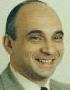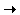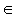# Cut The Knot!

An interactive column using Java applets
by Alex Bogomolny

# The Collage Theorem

March 1998That the terms of mathematics may be confusing is often claimed even by professional mathematicians. As an example, spaces and sets contain points. Yet there are spaces whose "points" are themselves sets. Functions map points to points but also comprise (function) spaces where each is considered an indivisible one. From the mathematician's view point, the advantage of abstracting a point from its diverse incarnations is in the achieved generality. A statement is proven only once and then applied repeatedly in various disguises.

Case in point - contraction mappings and the related convergence theorems. Contraction mappings are defined on metric spaces by the property that they decrease the distance between points. In complete spaces, any contraction mapping has a unique fixed point that can be found by (necessarily) convergent iterations. Convergence estimates relate proximity of iterations to the selection of the initial point. These general estimates apply as much to the solutions of integral and differential equations, matrices, and more general operators. Iterated Function Systems (IFSs) is another rewarding topic that capitalizes on the general theory.

An Iterated Function System is a finite collection of contractions Fi: XX defined on a metric space X. Each extends to a mapping (different but denoted by the same letter) Fi: H(X)H(X), where H(X) is the space whose points are nonempty compact subsets of X. When endowed with the Hausdorff metric, H(X) is complete if so was X. In addition, contractions FiXX remain contractions as mappings of H(X). Together, {Fi} define another contraction F: H(X)H(X), by the following formula: for every AH(X), F(A) = ∪Fi(A). From the general theory, for a complete metric space X, F has a fixed point AF: F(AF) = AF, that can be reached by successive approximations from any starting location. Fixed points of IFSs are variously called attractors or invariant sets.

Two problems arise. One is to find the fixed point of a given IFS. The other is the inverse of the first: for a given set AH(X), find an IFS {Fi} that has A as its fixed point. In the general framework, the first problem is solved by what is known as the deterministic algorithm. Start with a set A0∈H(X) and compute successively Ak = ∪Fi(Ak-1) = F(Ak-1), k > 1. The sequence {Ak) converges to the fixed point AF of {Fi} as k. The mathematics of the second, random iteration algorithm, is more complex but implementation is more straightforward. Assign positive frequencies pi to the mappings Fi. Start with an arbitrary point x0∈X. At every step k+1, select xk+1 from the set {Fi(xk)}. Fj(xk) is selected with the probability pj /pi. In a sense that is made precise in [Barnsley], the sequence {xk} converges to AF. In practical terms, to depict an approximation of AF on a computer, the points of the sequence are displayed starting with a reasonably large index. The numbers {pi} have no effect on the fixed point AF but influence significantly the rendering of its approximations.

The inverse problem is solved approximately by the Collage Theorem. In the words of M. Barnsley, the theorem tells us that to find an IFS whose attractor is "close to" or "looks like" a given set, one must endeavor to find a set of transformations - contraction mappings on a suitable set within which the given set lies - such that the union, or collage, of the images of the given set under transformations is near to the given set.

The applet below was inspired by Barnsley's celebrated depiction of the Black Spleenwort fern. Three parallelograms almost covering the fourth and bigger one looked as naturally associated with the fern as each of its smaller branches. There was another parallelogram - a smallish and a degenerate one - that is often omitted in reproductions.

Parallelograms lead to affine transformations; for the latter map parallel lines onto parallel lines. Affine transformations can be uniquely determined from images of any three distinct points - three consecutive vertices of a parallelogram in particular.

The applet consists of two parts. The first is the display area with several controls. First of all, there are several predefined IFSs to select from. For a given IFS, Start starts the iterations of the random iteration algorithm; Stop stops them. The applet attempts to optimally exploit the visual estate. The algorithm is heuristic and sometimes is off by an iteration or two. If needed, Shrink the emerging image and Clear the area. Often, the component sets Fi(AF) intersect only over sets of lower dimension. In such a case, the Color option, that randomly assigns a color to each Fi(AF), may create an unusually beautiful effect. Reset restores the original state of the IFS.

A simple observation that two IFSs, {Fi} and {FiFj} have exactly the same fixed point, leads to a representation of AF as a union of a growing number of sets. To see how this works use the Iterate button.

When the Transforms box is checked the second portion of the applet (Affine Basis Manipulator) appears in a separate window. This is the place to apply the Collage theorem. The Which buttons designate one of the parallelograms to manipulate. The selected parallelogram is displayed in red-blue-white colors whereas all others are entirely gray. Red and blue edges can be changed independently or the whole structure can rotate around their common vertex. The first parallelogram is the most basic. All others are considered its images under affine transformations. Please bear in mind that the number of transformations is, therefore, 1 less than the number of parallelograms.

Apply the Iterate button with the Affine Basis Manipulator on. The regions will multiply and become smaller. To boot, this is a demonstration of the deterministic algorithm with the first parallelogram as the starting point.

Each of the parallelograms is an image of the unit square (0,0)-(1,1) under an affine mapping (x,y)(ax+by+e, cx+dy+f). Two rows of edit controls give you a direct access to the values of a,b,e (the first row) and c,d,f (the second row.) Beware: the coordinate system is the usual one for computer graphics. Y axis grows downwards.

### This applet requires Sun's Java VM 2 which your browser may perceive as a popup. Which it is not. If you want to see the applet work, visit Sun's website at https://www.java.com/en/download/index.jsp, download and install Java VM and enjoy the applet.

 What if applet does not run?

Using parallelograms to define affine transformations may be a mixed blessing. For one, parallelograms may not resemble the resulting sets in the least. Even overlapping parallelograms may sometimes lead to a perfect tiling of the resulting set (see Sierpinski 2). Further, two parallelograms can be mapped onto each other in 8 different ways. (Pick a vertex as the origin and the order of the two adjacent vertices.) So, while a parallelogram remains the same geometric region, it can be associated with several transformations. Each such selection leads to a different IFS with a different fixed point. Bring up the second window and compare the Twindragon, Dragon, Monster, and Surprise IFSs. They all start with the same regions. But Iterate and watch the deterministic algorithm at work.

### References

1. M. Barnsley, Fractals Everywhere, Academic Press, 1988
2. Chaos and Fractals, R. L. Devaney and L. Keen (eds), AMS, 1989
3. D. M. Davis, The Nature and Power of Mathematics, Princeton University Press, 1993
4. P. Hilton, D. Holton, J. Pederson, Mathematical Reflections, Springer Verlag, 1997
5. The Science of Fractal Images, H.-O.Peitgen and D.Daupe (eds), Springer-Verlag, 1988

### On the Web

1. IFS Tools by L. Brin.